# 2nd PUC Chemistry Question Bank Chapter 7 The p-Block Elements

## Karnataka 2nd PUC Chemistry Question Bank Chapter 7 The p-Block Elements

### 2nd PUC Chemistry The p-Block Elements NCERT Textbook Questions and Answers

Question 1.
Discuss the general characteristics of Group 15 elements with reference to their electronic configuration, oxidation state, atomic size, ionisation enthalpy, and electronegativity.
The valence shell electronic configuration of group 15 elements is ns2np3. Due to half-filled p-orbitals, these elements have extra stability associated with them.

The common oxidation states of these elements are -3, +3 and +5. The tendency to exhibit -3 oxidation state decreases down the group. The stability of +5 state decreases and that of +3 state increases down the group due to inert pair effect.

The size of group 15 elements increases down the group. There is a considerable increase in covalent radius from N to P. However, from As to Bi only a small increase in covalent radius is observed. This is due to the presence of completely filled d and or f orbitals in heavier members.

Down the group, ionisation enthalpy decreases due to an increase in atomic size. Due to stable half-filled configuration, they have much greater value than group 14 elements.

The electronegativity value, in general, decreases down the group with increasing atomic size. However, amongst the heavier elements, the difference is not that much pronounced.

Question 2
Why does the reactivity of nitrogen differ from phosphorus?
Molecular nitrogen exists as a diatomic molecule having a triple bond between the two nitrogen atoms, N = N (due to it stability to form pπ-pπ multiple bonding). The bond dissociation energy is very high (941-4 kJ mol-1). Thus, under ordinary conditions, nitrogen behaves as an inert gas. On the other hand, white and yellow phosphorus exists as a triatomic molecule (P4) having single bonds. The dissociation energy of the P-P bond is low (213 kJ mol-1). Thus, phosphorus is much more reactive than nitrogen.

Question 3
Discuss the trends in chemical reactivity of group 15 elements.
General trends in chemical properties of group-15
(i) Reactivity towards hydrogen:
The elements of group 15 react with hydrogen to form hydrides of type EH3, where E – N, P, As, Sb, or Bi. The stability of hydrides decreases on moving down from NH3 to BiH3

(ii) Reactivity towards oxygen:
The elements of group 15 form two types of oxides: E2O3 arid E2O5, where E = N, P, As, Sb or Bi. The oxide with the element in higher oxidation state is more acidic than the other. However, the acidic character decreases on moving down a group.

(iii) Reactivity towards halogens:
The group 15 element react with halogens to form two series of salts: EX3 and EX5. However, nitrogen does not form NX5 as H lacks the d-orbital. All trihalides (except NX3) are stable.

(iv) Reactivity towards metals:
The group 15 elements react with metals to form binary compounds in which these metals exhibit-3 oxidation states.

Question 4
Why does NH3 form a hydrogen bond but PH3 does not?
The N – H bond in ammonia is quite polar as nitrogen is highly electronegative in nature. As a result, NH3 molecules are linked by intermolecular hydrogen bonding. On the other hand, P – H bond is non-polar as both P and H have the same electronegativity. Hence in phosphine, no hydrogen bonding is present.Question 5.
How is nitrogen prepared in the laboratory? Write the chemical equations of the reactions involved.
An aqueous solution of ammonium chloride is treated with sodium nitrite
NH4Cl + NaNO2(aq) → N2(g) + 2H2O(e) + NaCl(aq)
NO, and HNO3 are produced in small amounts. These are impurities that can be removed on passing nitrogen gas through aqueous H2SO4 containing potassium dichromate

Question 6
How is ammonia manufactured industrially?
Ammonia is prepared on a commercial scale by Haber’s Process from dinitrogen and dihydrogen by the following chemical reaction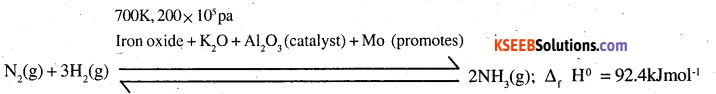Dihydrogen needed for the commercial preparation of ammonia is obtained by the electrolysis of water while dinitrogen is obtained from the liquefied air as fractional distillation. The two gases are purified and also dried.
These are compressed to about 200-atmosphere pressure and are then led into the catalyst chamber packed with the catalyst and the promoter.

Question 7
Illustrate how copper metal can give different products on reaction with HNO3.
On heating with dil HNO3, copper gives copper nitrate and nitric oxide.Question 8
Give the resonating structures of NO2 and N2O5.
Resonating structures of NO2 are: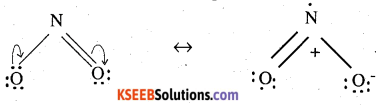Resonating structures of N2O5 are:Question 9.
The HNH angle value is higher than HPH, HAsH, and HSbH angles. Why?
[Hint: Can be explained on the basis of sp3 hybridisation in NH3 and only s-p bonding between hydrogen and other elements of the group].
Hydride NH3, PH3, AsH3, SbH3
H-M-H angle 107°, 92°, 91°, 90°
The central atom (E) in all the hydrides is sp3 hybridised. However, its electronegativity decreases, and atomic size increases down the group. As a result, there is a gradual decrease in the force of repulsion in the shared electron pairs around the central atom. This leads to a decrease in the bond angle.Question 10.
Why does R3P = O exist but R3N = O does not (R = alkyl group)?
Nitrogen does not have d-orbitals in its valence shell. Therefore, it can not extend its covalency to five by forming dπ-dπ bonding. As a result, the molecule of R3N = O does not exist. However, phosphorus has vacant rf-orbitals in the valence shell and can form dx-dx bonding. Thus, a molecule like R3P = O can exist.

Question 11.
Explain why NH3 is basic while BiH3 is only feebly basic.
Both NH3 and PH3 are Lewis bases due to the presence of lone electron pair on the central atom. However, NH3 is more basic than PH3. The atomic size of nitrogen (Atomic radius = 70 pm) is less than that of phosphorus (Atomic radius = 110 pm) As a result, electron density on the nitrogen atom is more than on phosphorus. This means that the electron releasing tendency of ammonia is also more and is, therefore, a stronger base than phosphine.

Question 12.
Nitrogen exists as a diatomic molecule and phosphorus as P4. Why?
Nitrogen because of its small size and high electronegativity is capable of forming pπ-pπ multiple bonding. Therefore, it exists as a diatomic molecule with one σ and two K bonds (N ≡ N). Phosphorus, on the other hand, has a longer size and lower electronegativity and thus is not capable to form pπ-pπ multiple bonds. It prefers to form single bonds hence, it exists as tetrahedral P4 molecules.

Question 13
Write the main differences between the properties of white phosphorus and red phosphorus.

 White phosphorous Red phosphorous It is a soft and waxy solid. It possesses a garlic smell. It is hard and crystalline solid. It is poisonous without any smell It is insoluble in water but soluble in carbon disulphide It is non-poisonous It is insoluble in both water and carbon It undergoes spontaneous combustion in air disulphide It is relatively In both solid and vapour states, it exists as a P4 molecule less reactive It exists as a chain of tetrahedral P4.

Question 14
Why does nitrogen show catenation properties less than phosphorus?
The extent of catenation depends upon the strength of the element-element bond. The N – N bond strength (159 kJ mol-1 ) is weaker than the P – P bond strength (213 kJ mol-1 ). Thus, nitrogen shows fewer catenation properties than phosphorus.

Question 15
Give the disproportionation reaction of H3PO3.
H3PO3 on heating undergoes self oxidation-reduction, i.e. disproportionation to form PH, in which P is reduced, and H3PO4 in which P is oxidised.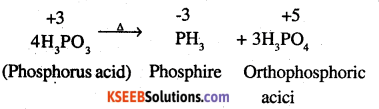Question 16.
Can PCl5 act as an oxidising as well as a reducing agent? Justify.
Phosphorus has a maximum oxidation state of +5 in PCl5. It can not increase its oxidation state further and thus it can not act as a reducing agent. However, PCl5 can act as an oxidising agent as it can itself reduce from +5 to +3 oxidation state.

Question 17.
Justify the placement of Q, S, Se, Te, and Po in the same group of the periodic table in terms of electronic configuration, oxidation state, and hydride formation.
The elements of group 16 are collectively called chalcogens.
(i) Elements of group 16 have six valence electrons each. The general electronic configuration of these elements is ns2 np4, where n varies from 2 to 6.

(ii) oxidation state:
These elements have six valence electrons (ns2 np4). They should display an oxidation state of -2. However only oxygen predominantly shows the oxidation state of -2 owing to its high electronegativity. It also exhibits an oxidation state of -1 (H2O2), Zero (O2), and +2 (OF2) However, the stability of the -2 oxidation state decreases on moving down a group due to a decrease in the electronegativity of the elements. The heavier elements of the group show an oxidation state of +2, +4 and +6 due to the availability of d-orbitals.

(iii) Formation of hydrides
These elements form hydrides of formula H2E, where E = O, S, Se, Te, PO. Oxygen and sulphur also form hydrides of type H2E2. These hydrides are quite volatile in nature.Question 18.
Why is dioxygen a gas but sulphur a solid?
The oxygen atom has the tendency to form multiple bonds (pπ – pπ interaction) with other oxygen atoms on account of small size while this tendency is missing in sulphur atom. The bond energy of oxygen-oxygen double bond (0 = 0) is quite large (about three times that of an oxygen-oxygen single bond, O – O = 34.9 kcal mol-1) while sulfur-sulfur double bond (S = S) is not so large (less than double of sulphur-sulphur single bond, S – S = 63.8 kcal mob1). As a result —O—O—O— chains are less stable as compared to O = O molecule while —S—S—S— chains are more stable than S = S molecule. Therefore, at room temperature, while oxygen exists as a diatomic gas molecule, sulphur exists as S8 solid.

Question 19.
Knowing the electron gain enthalpy values for O → O and O → O2- as -141 and 702 KJ mol-1 respectively, how can you account for the formation of a large number of oxides having O2- species and not O?
(Hint: Consider lattice energy factor in the formation of compounds).
According to available data :
O + e → O; ∆(eg) H = – 141 kJ mol-1
O + 2e → O2-; ∆(eg) H = + 702 kJ mol -1
Although the formation of the divalent anion (O2-) needs more energy as compared to the monovalent anion (O) where energy is actually released, still in a large number of oxides (e.g Na2O, K2O, CaO etc.) oxygen is divalent in nature. This is on account of a more stable crystal lattice because of the greater magnitude of electrostatic forces of attraction involving divalent oxygen than the oxides in which oxygen is monovalent in nature.

Question 20
Which aerosols deplete ozone?
Freon (CCl2F2) (Chlorofluoro carbon).

Question 21.
Describe the manufacture of H2SO4 by contact process?
H2SO4 is manufactured by contact process. It involves the following steps

Step (1)
Sulphur or sulphide ores are burnt in the air to form SO2.

Step (2)
By a reaction with O2, SO2 is converted to SO3 in the presence of V2O5 as a catalyst.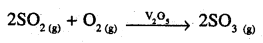Step (3)
SO3 produced is absorbed on H2SO4 to give H2S2O7(Oleum)

SO3+ H2SO4 → H2S2O7

This oleum is then diluted to obtain H2SO4, of the desired concentration. In practice, the plant is operated at 2 bar (pressure) and 720 K (temperature). The H2SO4 thus obtained is 96 – 98% pure.

Question 22.
How is SO2 an air pollutant?
(i) Sulphur dioxide released in the atmosphere during the combustion of fuels combines with H20 molecules and oxygen present to form sulphuric acid. The acid being poisonous in
SO2 + 1/2O2 + H2O → H2SO4
nature causes pollution. It causes the corrosion of statues and monuments made from marble (CaC03) due to the formation of sulphate.
CaCO3 + H2SO4 → CaSO4 + H2O + CO2
(ii) Sulphur dioxide adversely affects the respiratory tract due to its poisonous as well as irritating nature. It causes throat infection as well as irritation in the eyes.
(iii) Even a very low concentration of gas (0·03 ppm) has a very damaging effect on plants and vegetation. This is called chlorosis. It slows down the formation of chlorophyll and the leaves slowly wither.

Question 23.
Why are halogens strong oxidising agents?
The halogens are strong oxidising agents due to low bond dissociation enthalpy, high electronegativity, and large negative electron gain enthalpy.Question 24.
Explain why fluorine forms only one.
Fluorine forms only one oxoacid, i.e. HOF because of its high electronegativity and small size.

Question 25
Explain why in spite of nearly the same electronegativity, oxygen forms hydrogen bonding while chlorine does not.
Both nitrogen (N) and chlorine (Cl) have electronegativity of 3·0. However, only nitrogen is involved in the hydrogen bonds (e.gNH3) and not chlorine. This is an account of the smaller atomic size of nitrogen (atomic radius = 70 pm) as compared to chlorine (atomic radius = 99 pm). Therefore, N can cause greater polarisation of N—H bond than Cl in case of Cl—H bond. Consequently, the N atom is involved in hydrogen bonding and not chlorine.

Question 26
Write two uses of ClO2.
Two uses of ClO2:

• It is a powerful oxidising and chlorinating agent.
• It is an excellent bleaching agent for wood pulp, flour for making white bread.

Question 27
Why are halogens coloured?
Almost all halogens are coloured. This is because halogens absorb radiations in the visible region. This results in the excitation of valence electrons to a higher energy region. Since the amount of energy required for excitation differs for each halogen, each halogen displays a colour.

Question 28
Write the reactions of F2 and Cl2 with water.Question 29
How can you prepare Cl, from HCl and HCl from Cl2? Write reactions only.
Cl2 can be prepared from HC1 by Deacon’s process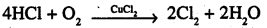(ii) HCl can be prepared from Cl by treating it with water
Cl2 + H2O → HCl + HOCl

Question 30
What inspired N. Bartlett for carrying out a reaction between Xe and PtF6?
Neil Bartlett observed that PtF6 reacts with O2 to form an ionic solid O+2 PtF6
O2(g) + PtF6(g) → O+2[PtF6]
In this reaction, O2 gets oxidised to O+2 by PtF6.
Since the first ionisation energy of xenon is fairly close to that of oxygen. Bartlett thought that PtF6 should also oxidise Xe to Xe+. This prompted Bartlett to carry out the reaction between Xe and PtF6 and formed the first noble gas compound.

Question 31.
What are the oxidation states of phosphorus in the following:
(i) H3PO3
(ii) PCl3
(iii) Ca3P2
(iv) Na3PO4
(v) POF3?
Let oxidation state of P be x
(i) H3PO3
3 + x + 3 (-2) = 0
3 + x – 6 = 0
x = 3.

(ii) PCl3
x + 3 (-1) = 0
x – 3 = 0
x = 3

(iii) Ca3P2
3(2) + 2x = 0
6 + 2x = 0
x = -3

(iv) Na3PO4
3 x 1 +x + 4(-2) = 0
3 + x – 8 = 0
x = 5

(v) POF3
x + (-2) + 3x (-1) = 0
x – 5 = 0
x = 5.

Question 32.
Write balanced equations for the following:
(i) NaCl is heated with sulphuric acid in the presence of MnO2.
(ii) Chlorine gas is passed into a solution of Nal in water.
(i) Cl2 is produced
4NaCl + MnO2 + 4H2SO4 → MnCl2 + 4NaHSO4 + 2H2O + Cl2
(ii) Cl2 being an oxidising agent oxidises Nal to I2.
Cl2(g) + 2NaI(aq) → 2NaCl(aq) + I2(s)Question 33.
How are xenon fluorides XeF2, XeF4 and XeF. obtained?
XeF2, X3F4 and XeF6 are obtained by a direct reaction between Xe and F2. The condition under which the reaction is carried out determines the product.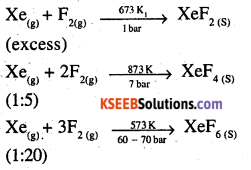Question 34
With what neutral molecule’ is ClO isoelectronic? Is that molecule a Lewis base?
ClO has 17 + 8 +1 = 26 electrons.Also, OF2 has (8 + 2 x 9) = 26 electrons, and ClF has (17 + 9) = 26 electrons.Out of these, ClF can act as Lewis base. The chlorine atom has three lone pair of electrons which it donates to form compounds like ClF3, ClF5 and ClF7

Question 35
How are XeO3 and XeOF4 prepared?
(i) XeO3 can be prepared in two ways as shown:
6XeF4 + 12H2O → 4Xe + 2XeO3 + 24HF + 3O2 XeF6 + 3H2O → XeO3 + 6HF
(ii) XeF4 can be prepared using XeF6
XeF6+ H2O → XeOF4 + 2HF

Question 36.
Arrange the following in the order of property indicated for each set :
(i) F2, Cl2, Br2, I– increasing bond dissociation enthalpy.
(ii) HF, HCl, HBr, HI – increasing acid strength.
(iii) NH3, PH3, AsH3, SbH3, BiH3 – increasing base strength. (Pb. Board 2009, C.B.S. Sample Paper 2017)
(i) I2 (151-1 kJmol-1) < F2 (158-8 kJ mol-1) < Br2 (192-8 kJ mol-1) < Cl2 (242-6 kJ mol-1)
(ii) HF < HCl < HBr < HI
(iii) BiH3 < SbH3 < ASH3 < PH3 < NH3.Question 37.
Which one of the following does not exist?
(i) XeOF4
(ii) NeF2
(iii) XeF2
(iv) XeF
(ii) NeF2

Question 38.
Give the formula and describe the structure of a noble gas species which is isostructural with:
(i) ICl4
(ii) IBr2
(iii) BrO3
(i) XeF4 is isoelectronic with ICl4 and has square planar geometry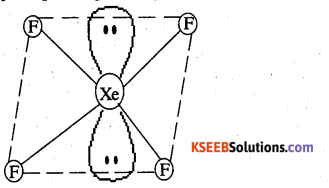(ii) XeF2 is isoelectronic to IBr2 and has a linear structure(iii) XeO3 is isostructural to BrO3 and has a pyramidal molecular structure.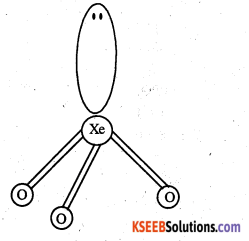Question 39.
Why do noble gases have comparatively large atomic sizes?
This is because noble gases have only van der Waal’s radii while others have covalent radii, van der Waal’s radii are larger than covalent radii.Question 40
List the uses of neon and argon gases.
Uses of Neon:

• Neon lamps are used in botanical gardens and also in greenhouses as they are useful in the formation of chlorophyll and thus, stimulate plant growth.
• It is used in filling sodium vapour lamps.
• It is used in safety devices for protecting certain electrical instruments (voltmeters, relays, rectifiers etc.)

Uses of Argon:

• It is used in metal filament electric lamps since it increases the life of the tungsten filament by retarding its vapourisation.
• A mixture of argon and mercury vapours is used in fluorescent tubes.
• It is used to create an inert atmosphere for welding and for carrying certain chemical reactions.

Question 1.
What is the electronic .configuration of p-block elements?
ns2np0 – 5

Question 2.
Write three uses of nitrogen?

1. Used in the manufacture of nitric acid, ammonia, calcium cyanamide.
2. The inert atmosphere for the iron and steel industry.
3. It is used as a refrigerant.

Question 3.
Write three physical properties of Ammonia?

1. It is a colourless gas.
2. It is lighter than air.
3. Extremely soluble in water.

Question 4.
Write three physical properties of Nitric acid?

1. Colourless fuming liquid.
2. The melting point is 231.4k.
3. The boiling point is 355.6 k.

Question 5.
What are the three allotropic forms of phosphorous?

1.  white phosphorous
2. Red phosphorous
3. Black phosphorous.

Question 6.
Write the chemical formula of Hypo phosphorus acid.
H3PO2.Question 7.
Write the chemical formula of orthophosphorous acid.
H3PO3

Question 8.
Write the chemical formula of pyrophosphoric acid.
H4P2O5

Question 9.
Draw the structure of PCl5.